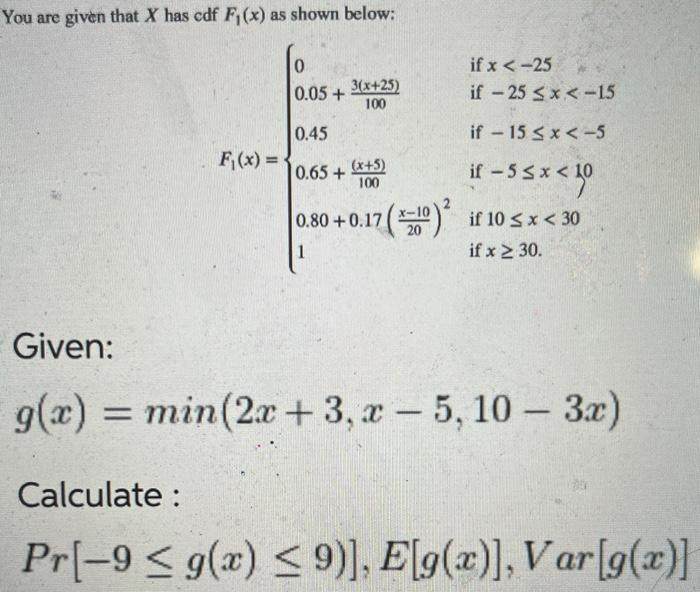Home / Expert Answers / Statistics and Probability / you-are-given-that-x-has-cdf-f-1-x-as-shown-below-f-1-x-left-begin-array-pa809

# (Solved): You are given that $$X$$ has cdf $$F_{1}(x)$$ as shown below: $F_{1}(x)=\left\{\begin{array}{ ...You are given that $$X$$ has cdf $$F_{1}(x)$$ as shown below: \[ F_{1}(x)=\left\{\begin{array}{ll} 0 & \text { if } x<-25 \\ 0.05+\frac{3(x+25)}{100} & \text { if }-25 \leq x<-15 \\ 0.45 & \text { if }-15 \leq x<-5 \\ 0.65+\frac{(x+5)}{100} & \text { if }-5 \leq x<10 \\ 0.80+0.17\left(\frac{x-10}{20}\right)^{2} & \text { if } 10 \leq x<30 \\ 1 & \text { if } x \geq 30 \end{array}\right.$ Given: $g(x)=\min (2 x+3, x-5,10-3 x)$ Calculate : $\operatorname{Pr}[-9 \leq g(x) \leq 9)], E[g(x)], \operatorname{Var}[g(x)]$

We have an Answer from Expert